Previous Page | Next Page

 Module Library

## REGRESS Call

performs regression analysis

RUN REGRESS(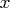,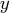, name, <tval>, <l1>, <l2>, <l3>);

The inputs to the REGRESS subroutine are as follows:is an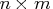numeric matrix, where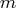is the number of variables and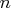is the number of data points.is an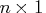response vector.

name
is an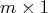matrix of variable names.

tval
is an optional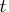-value.

l1, l2, l3
are optional
vectors that specify linear combinations of coefficients for hypothesis testing.
The REGRESS module does regression analysis and prints results. The design matrix is given by, andis the response vector. The name vector identifies each of the variables. If you specify a-value, the module prints a table of observed and predicted values, residuals, hat diagonal, and confidence limits for the mean and predicted values. If you also specify linear combinations with l1, l2, and l3, the module performs the hypothesis test
, where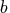is the vector of parameter estimates.Previous Page | Next Page | Top of Page